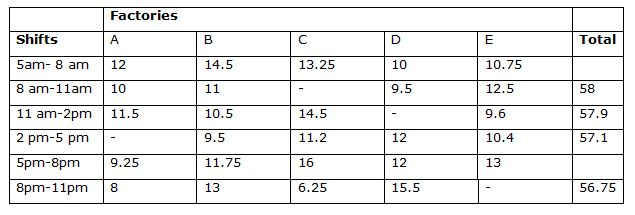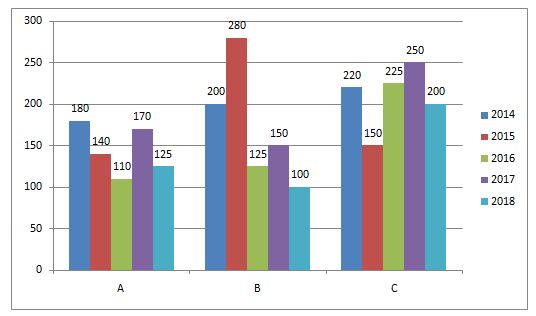# Quantitative Aptitude Questions (Data Interpretation) for IBPS RRB Assistant Prelims 2018 Day-162

Dear Readers, IBPS RRB is conducting Online Examination for the recruitment of clerical cadre To enrich your preparation here we have providing new series of Data Interpretation – Quantitative Aptitude Questions. Candidates those who are appearing in IBPS RRB Assistant Prelims Exams can practice these Quantitative Aptitude average questions daily and make your preparation effective.

Click “Start Quiz” to attend these Questions and view Solutions

Directions (1-5): Study the following table and answer the following questions.

The following table shows that the total number of employees in the given 6 shifts of various Companies (in thousands)1) What is the difference in the total number of employees working in various shifts in company C and the total number of employees working in various shifts in company D?

a) 5570

b) 2480

c) 5180

d) 5400

e) 2470

2) The total number of employees from Company A is approximately what percent of the total number of employees from company B ?

a) 63.17%

b) 92.17%

c) 86.17%

d) 32.17%

e) 35.17%

3) What is the total of the average number of employees working in the fourth shift and sixth shift in all the companies?

a) 23150

b) 22460

c) 22770

d) 24620

e) 25640

4) What is the total number of female employees working in various shifts in company E if the ratio of male and female employees in the company E is 2:3?

a) 44025

b) 44031

c) 44052

d) 42150

e) 44352

5) What is the ratio of the total number of employees from company B and company D working in the shift 8 am-11 am and the total number of workers working in the same shift in company  C and company E together?

a) 41:53

b) 41:55

c) 41:24

d) 32:55

e) 41:52

Directions (6-10): Study the following charts and answer the given questions.

The following bar graph shows that the number of students studying in branches A, B and C in a particular college from 2014 to 2018.6) By what percentage is the total number of students studying in branch B greater than those in branch C in the year 2015?

a) 32.67%

b) 86.67%

c) 93.67%

d) 64.67%

e) 54.67%

7) What is the ratio between the total students in branch A from 2016 to 2018 to the total students in branch C for 2014 and 2016?

a) 83:78

b) 52:75

c) 67:82

d) 63:89

e) 81:89

8) What is the difference in percentage change in the number of students of branch A and branch C in the year 2018?

a) 8.3%

b) 6.2%

c) 7.2%

d) 6.47%

e) 2.73%

9) In the year 2019, the number of students in branches A, B and C increases by 10%, 12.5% and 15% respectively from the year 2014, what is the difference between the total number of students across branches in 2019 and 2017?

a) 179

b) 163

c) 180

d) 106

e) 633

10) What is the percentage change in the number of students of branch B for the year 2017 as compared to 2016?

a) 25%

b) 20%

c) 35%

d) 15%

e) 50%

Direction (1-5)

Number of employees working in second shift of company C

=58 (10+11+9.5+12.5)                                                                                                  = (58-43) thousand

= 15 thousand

Total number of employees working in company C

= (13.25+15+14.5+11.2+16+6.25)=76.2

Now, Number of employees working in third shift of company D

=57.9-(11.5+10.5+14.5+12.5)

= 11.8 thousand

Total number of employees working in company D

= (10+9.5+11.8+12+12+15.5) =70.8

Required difference= 76.2-70.8=5.4 thousand

= 5400

Number of employees working in 2 pm- 5 pm in company A

= 57.1-43.1

= 14 thousand

Total number of employees working in company A= (12+10+11.5+14+9.25+8)

= 64.75

Total number of employees working in company B =14.5+11+10.5+9.5+11.75+13=70.25

Required percentage= (64.75/70.25)*100=92.17%

Average number of employees working in the fourth shift

= 57.1/5

= 11.42 thousand

= 11420

Average number of employees working in the sixth shift

= (56.75)/5

= 11.35 thousand

= 11350

Required total= 11420+11350=22770

In company E, number of employees working in sixth shift

=56.75 – (8+13+6.25+15.5) = 14 thousand

Total number of employees in company E

= 10.75+12.5+9.6+10.4+13+14                                                                           =70.25

Given that the ratio of male and female employees in Company E= 2:3

Then, we can say that, 2x+3x=70.25 thousand

(i.e)  5x= 70250

x= 14050

Then Number of female employees in the company E= 3x= 42150

Number of employees from company B and company D working in the shift 8 am-11 am=11+9.5=20.5

Number of workers working in the same shift in company C and company E=15+12.5=27.5

Required ratio=20.5:27.5

=41:55

Direction (6-10)

In 2015, Number of students in branch B= 280 and Number of students in branch C= 150

Hence required percentage= [(280-150)/150]*100

= 86.67%

Total number of students in branch A from 2016 to 2018= 125+170+110

= 405

Total number of students in branch C from 2014 and 2016= 225+220

= 445

Required ratio= 405:445

= 81:89

Percentage change for branch A in the year 2018 compared to 2017

= [(170-125)/170]*100

Percentage change for branch C in the year 2018 compared to 2017

= [(250-200)/250]*100

= 26.47%

Required difference= 26.47 – 20 = 6.47%

Total number of students in 2019

= (110% of 180) + (112.5% of 200) + (115% of 220)

= 198+225+253

= 676

Now total students in 2017= 170+150+250

= 570

Thus required difference=676- 570

= 106

Number of students in branch B in the year 2017=150

And Number of students in branch B in the year 2016=125

Thus required percentage change=[(150-125)/125]*100

= 20%

Daily Practice Test Schedule | Good Luck

 Topic Daily Publishing Time Daily News Papers & Editorials 8.00 AM Current Affairs Quiz 9.00 AM Quantitative Aptitude “20-20” 11.00 AM Vocabulary (Based on The Hindu) 12.00 PM General Awareness “20-20” 1.00 PM English Language “20-20” 2.00 PM Reasoning Puzzles & Seating 4.00 PM Daily Current Affairs Updates 5.00 PM Data Interpretation / Application Sums (Topic Wise) 6.00 PM Reasoning Ability “20-20” 7.00 PM English Language (New Pattern Questions) 8.00 PM